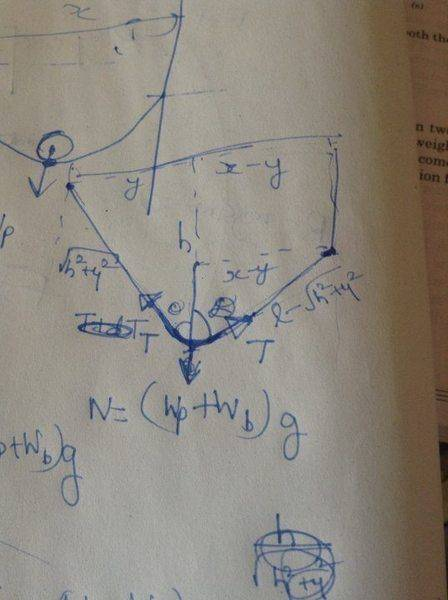# Equilibrium Tension Along a Curved String## Homework Statement

A light inextensible string of length l hangs on two pegs attached to parallel walls separated by a height. A small frictionless pulley of weight Wp is attached to a block of weight Wb. When it is placed on the string, the string becomes taut and pulley runs on the string till equilibrium is established. What is the tension in the string?

## Homework Equations

F=ma; vertically and horizontally
x, l, Wp, Wb are the given variables

## The Attempt at a Solution

The angles the tension force makes with the pulley at the point of contact are equal as the horizontal components of tension are equal. When equating the vertical component to mg, I only get an expression for T in terms of an unknown variable h. I eliminated y by using the two triangles marked in the diagram. Any thoughts?

What is the length between two walls?

haruspex